Processing ......FreeComputerBooks.com Links to Free Computer, Mathematics, Technical Books all over the World

Mathematics for Machine Learning
🌠 Top Free JavaScript Books - 100% Free or Open Source!
• Title Mathematics for Machine Learning
• Author(s) Marc Peter Deisenroth, A. Aldo Faisal, Cheng Soon Ong
• Publisher: Cambridge University Press; 1st edition (April 23, 2020); eBook (GitHub Edition)
• Permission: This PDF version is free to view and download for personal use only. Not for re-distribution, re-sale, or use in derivative works.
• Paperback: 398 pages
• eBook: PDF (412 pages)
• Language: English
• ISBN-10/ASIN: 110845514X
• ISBN-13: 978-1108455145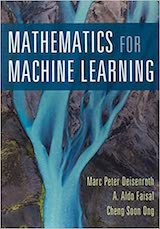Book Description

The fundamental mathematical tools needed to understand machine learning include linear algebra, analytic geometry, matrix decompositions, vector calculus, optimization, probability and statistics.

This self contained textbook bridges the gap between mathematical and machine learning texts, introducing the mathematical concepts with a minimum of prerequisites.

• Marc Peter Deisenroth is DeepMind Chair in Artificial Intelligence at the Department of Computer Science, University College London.
Reviews, Ratings, and Recommendations: Related Book Categories: Read and Download Links:Similar Books:
•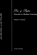Pen and Paper Exercises in Machine Learning (Michael Gutmann)

This is a collection of (mostly) pen-and-paper exercises in machine learning. The author assumes basic calculus, linear algebra, probability and statistics but no prior exposure to machine learning.

•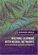Machine Learning with Neural Networks (Bernhard Mehlig)

This modern and self-contained book offers a clear and accessible introduction to the important topic of machine learning with neural networks. It provides comprehensive coverage of neural networks, their evolution, their structure, their applications, etc.

•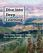Dive into Deep Learning (Aston Zhang, et al.)

This is an open source, interactive book provided in a unique form factor that integrates text, mathematics and code, now supports the TensorFlow, PyTorch, and Apache MXNet programming frameworks, drafted entirely through Jupyter notebooks.

•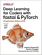Deep Learning for Coders with Fastai and PyTorch

This book show you how to train a model on a wide range of tasks using fastai and PyTorch. You'll also dive progressively further into deep learning theory to gain a complete understanding of the algorithms behind the scenes.

•Mathematics for Computer Science (Eric Lehman, et al)

This book covers elementary discrete mathematics for computer science and engineering. It emphasizes mathematical definitions and proofs as well as applicable methods. explores the topics of basic combinatorics, number and graph theory, logic and proof techniques.

•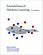Foundations of Machine Learning (Mehryar Mohri, et al)

This book is a general introduction to machine learning. It covers fundamental modern topics in machine learning while providing the theoretical basis and conceptual tools needed for the discussion and justification of algorithms.

•Understanding Machine Learning: From Theory to Algorithms

This book explains the principles behind the automated learning approach and the considerations underlying its usage. It provides an extensive theoretical account of the fundamental ideas underlying machine learning and the mathematical derivations.

•The Hundred-Page Machine Learning Book (Andriy Burkov)

Everything you really need to know in Machine Learning in a hundred pages! This book provides a great practical guide to get started and execute on ML within a few days without necessarily knowing much about ML apriori.

Book Categories
 :All CategoriesTop Free BooksRecent BooksMiscellaneous BooksComputer EngineeringComputer LanguagesComputer ScienceData Science/DatabasesJava and Java EE (J2EE)Linux and UnixMathematicsMicrosoft and .NETMobile ComputingNetworking and CommunicationsSoftware EngineeringSpecial TopicsWeb Programming
Other Categories